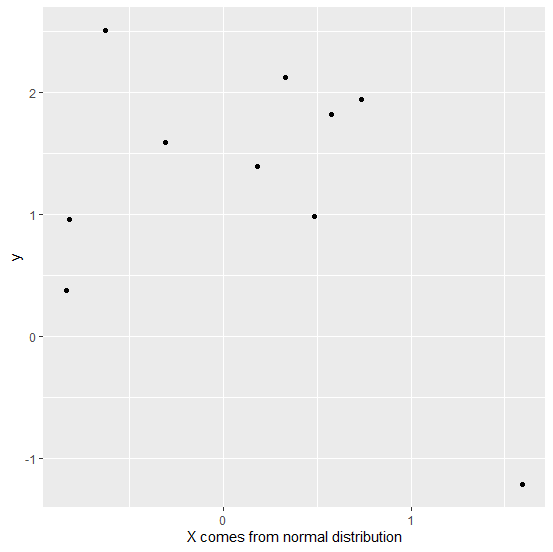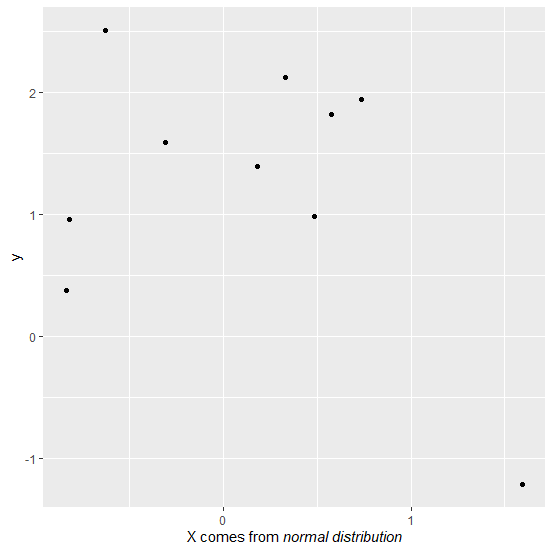# How to write partial title of X-axis in italics using ggplot2 of R?

Of course, writing axes titles help viewers to understand the plot in a better way because they add more information to the plot. In general, the axes titles have simple font but we can change partial or complete title to italics to get the viewers attraction. This is needed when we want to highlight the title by making it different. In ggplot2, we can do this by using expression.

## Example

Consider the below data frame −

set.seed(1)
x<-rnorm(10)
y<-rnorm(10,1)
df<-data.frame(x,y)
df

## Output

      x       y
1 -0.6264538  2.5117812
2  0.1836433  1.3898432
3 -0.8356286  0.3787594
4  1.5952808 -1.2146999
5  0.3295078  2.1249309
6 -0.8204684  0.9550664
7  0.4874291  0.9838097
8  0.7383247  1.9438362
9  0.5757814  1.8212212
10 -0.3053884 1.5939013
> library(ggplot2)

Creating the scatterplot between x and y −

ggplot(df,aes(x,y))+geom_point()+
+ labs(x=expression(paste("X comes from normal distribution")))

## OutputNow suppose we want to write normal distribution in italics then it can be done as shown below −

ggplot(df,aes(x,y))+geom_point()+labs(x=expression(paste("X comes from ",italic("normal distribution"))))

## Output